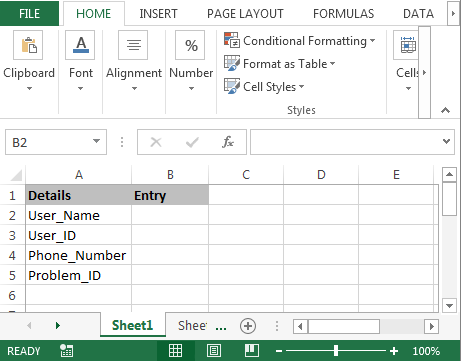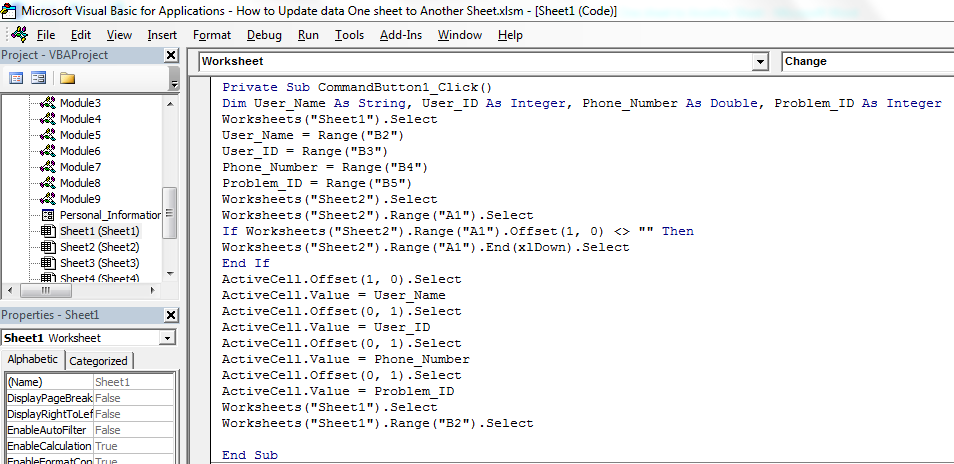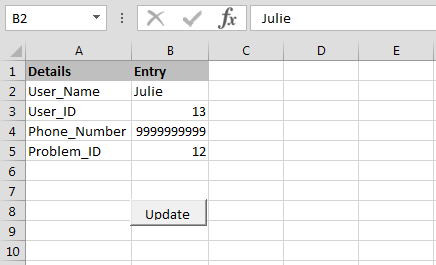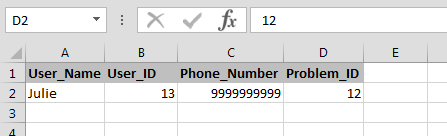# Update Sheets through VBA

In this article, we are going to learn how to update the data from one sheet to another sheet through VBA in Microsoft Excel.

Let’s take an example to understand how and where we can use this Macro in our data to reduce our daily work.

We want to write this code for those employees who are working in a call center. When clients call them they ask for basic details like User_Name, User_ID, Phone_Number, and Problem_ID, they need such a code to work systematically.

Now we want to apply the macro for such a context when a call comes and they note down all the above mentioned details into an excel sheet, and when they click on update, that data should be updated in another sheet.To update the data from one sheet to another sheet, follow below given steps:-

• First, we will insert command button in the worksheet.
• Go to Developer tab and then insert Command button from Activexcontrol.
• Assign below mentioned macro:-

Private Sub CommandButton1_Click()

Dim User_Name As String, User_ID As Integer, Phone_Number As Double, Problem_ID As Integer

Worksheets("Sheet1").Select

User_Name = Range("B2")

User_ID = Range("B3")

Phone_Number = Range("B4")

Problem_ID = Range("B5")

Worksheets("Sheet2").Select

Worksheets("Sheet2").Range("A1").Select

If Worksheets("Sheet2").Range("A1").Offset(1, 0) <> "" Then

Worksheets("Sheet2").Range("A1").End(xlDown).Select

End If

ActiveCell.Offset(1, 0).Select

ActiveCell.Value = User_Name

ActiveCell.Offset(0, 1).Select

ActiveCell.Value = User_ID

ActiveCell.Offset(0, 1).Select

ActiveCell.Value = Phone_Number

ActiveCell.Offset(0, 1).Select

ActiveCell.Value = Problem_ID

Worksheets("Sheet1").Select

Worksheets("Sheet1").Range("B2").Select

End Sub

Code Explanation:-First, we had defined all the variables. Then we had defined the range for every variable, and then described the range for all the variables. And, we had also defined that where and in which range data will be updated after clicking on Update button.• To run the code, press key F5 on the keyboard.
• When you will enter the data and will click on update button then data will be updated in another sheet and we can enter new data in the sheet.This is the way we can update the data from one sheet to another sheet through VBA in Microsoft excel.Download - How to Update data One sheet to Another Sheet - xlsm

1.